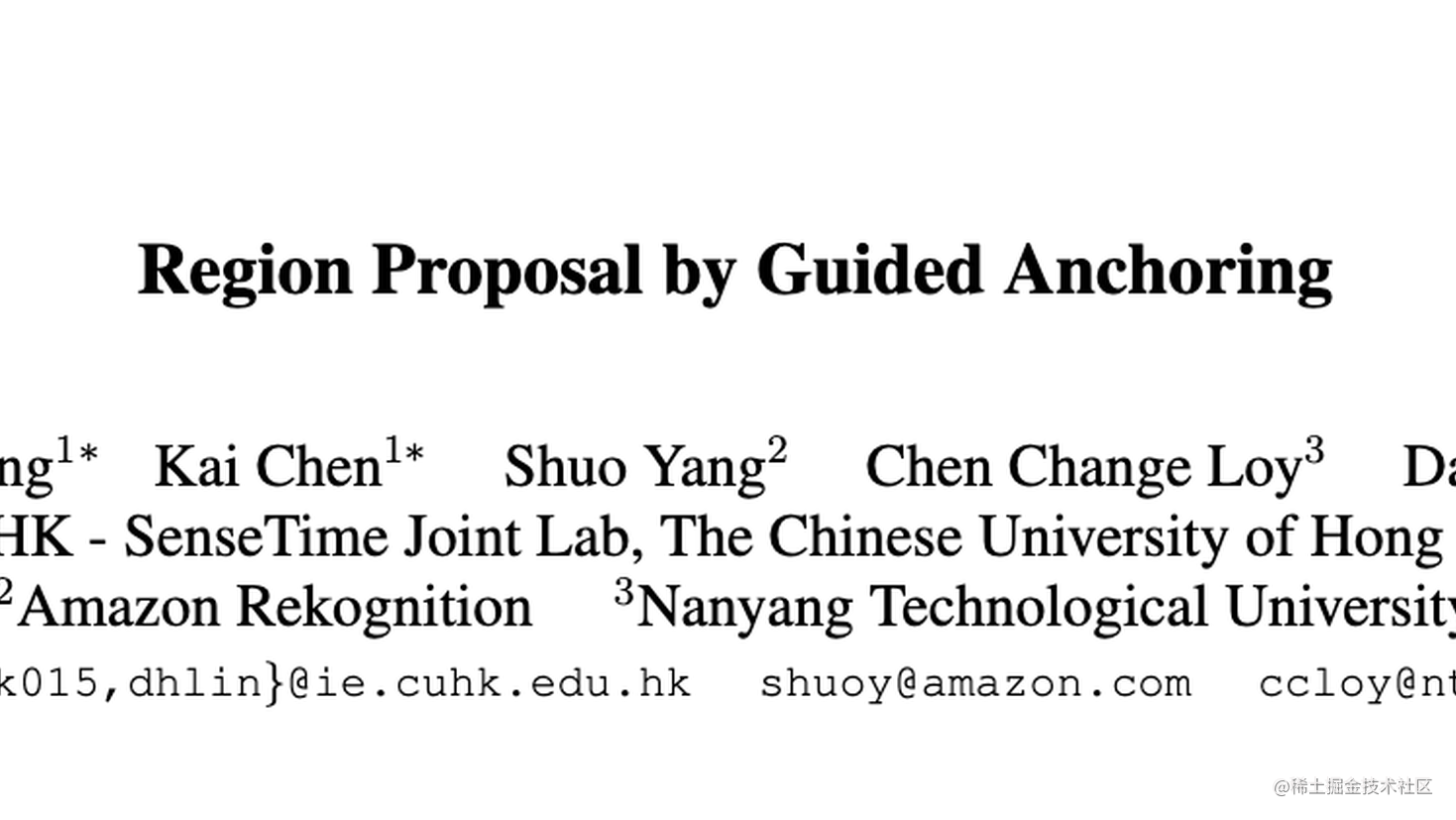# Guided Anchoring：在线稀疏anchor生成方案，嵌入即提2AP | CVPR 2019

Guided Anchoring通过在线生成anchor的方式解决常规手工预设anchor存在的问题，以及能够根据生成的anchor自适应特征，在嵌入方面提供了两种实施方法，是一个很完整的解决方案

来源：晓飞的算法工程笔记 公众号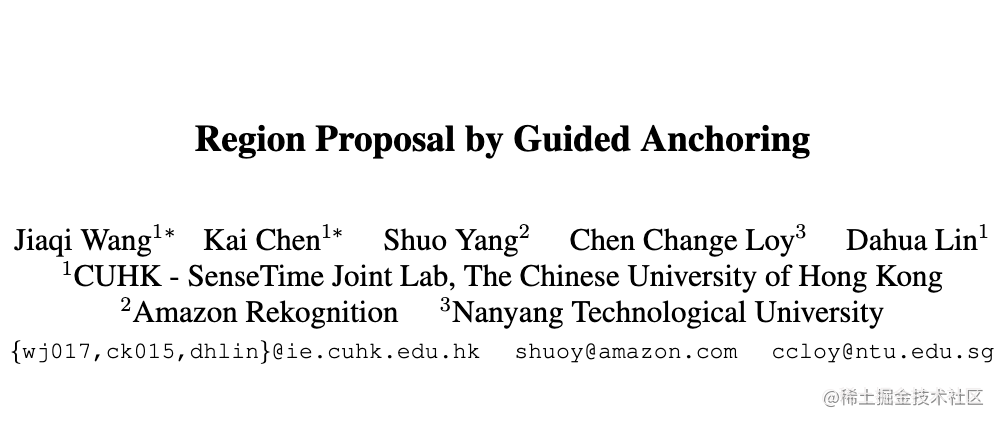# Introduction

Anchor是许多目标检测算法中一个很重要的机制，但也会带来两个问题：

• 需要预先设计合适的anchor尺寸和长宽比，如果设计不好，对速度和准确率造成很大影响
• 为了使anchor达到足够的召回率，需要在特征图上铺设大量的anchor，不仅引入大量负样本，还耗费计算量

为此，论文提出Guided Anchoring来根据图片特征在线生成anchor。首先判断目标可能出现的位置，然后学习不同位置上的目标的形状，可根据图片特征在线学习稀疏的候选anchor。然而，在线生成的anchor形状各异，固定的感受域可能不匹配其形状，所以Guided Anchoring根据anchor的形状进行自适应特征提取，然后再进行预测框精调与分类。

# Guided Anchoring

Guided Anchoring尝试在线学习不同形状的anchor及其位置，得到在特征图上不均匀分布的anchor集合。目标可用四元组$(x,y,w,h)$表示，其位置和形状可认为是服从图像$I$的一种分布：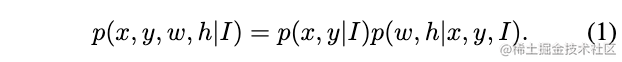公式1包含两部分：1) 给定图片，目标仅存在于某些区域 2) 形状与位置紧密相关。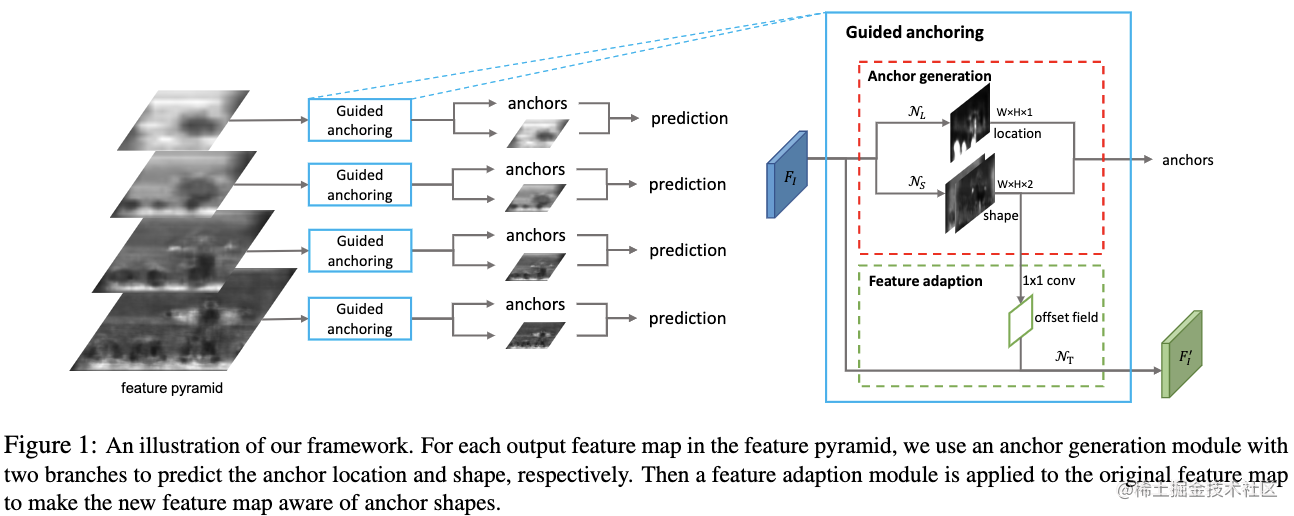基于公式1，论文设计了图1的anchor生成器(Anchor generation)，包含位置预测和形状预测两个分支。给定图片$I$，首先得到特征图$F_I$，位置预测分支根据$F_I$预测该像素为目标位置的概率，形状预测分支则预测像素位置相关的形状，基于两个分支选择概率高于阈值以及每个位置最合适的anchor，得到最终的anchor集合。由于集合中的anchor形状可能差异很大，每个位置需要获取不同大小区域的特征，论文提出特征自适应模块(Feature adaption)，根据anchor形状自适应提取特征。   上述的生成过程是基于单个特征的，网络的整体架构包含FPN，所以为每层都配备Guided anchoring模块，模块参数是层间共享的。

### Anchor Location Prediction

位置预测分支预测特征图$F_I$的概率图$p(\cdot|F_I)$，每项$p(i,j|F_I)$为该位置是目标中心的概率，对应的输入图片中坐标$((i+\frac{1}{2})s, (j+\frac{1}{2})s)$$s$为特征图的stride。   在实现时，通过子网$\mathcal{N}_L$进行概率图预测，首先使用$1\times 1$卷积提取主干网络特征图$F_I$的objectness分数，再通过element-wise sigmoid函数转化概率。更复杂的子网可以带来更高的准确率，论文采用的是准确率和速度最实惠的结构，最后取高于阈值$\epsilon_L$的像素位置，能够保证在高召回的前提下过滤90%的无关区域。

### Anchor Shape Prediction

形状预测分支的目标是预测每个位置对应目标的最佳形状$(w,h)$，但由于过大的数值范围，如果直接预测其具体数字会十分不稳定，所以先进行转化：形状预测分支输出$dw$$dh$，根据公式2转换出形状$(w,h)$$s$为特征图的stride，$\sigma=8$为人工设置的缩放因子。这个非线性转换可将[0, 1000]映射到[-1, 1]，更容易学习。在实现时，通过子网$\mathcal{N}_S$进行形状预测，首先使用$1\times 1$卷积获得两维特征图，分别对应$dw$$dh$，然后通过公式2进行转换。由于每个位置的anchor都是学习来的，与预设的固定anchor对比，这种学习的anchor召回率更高。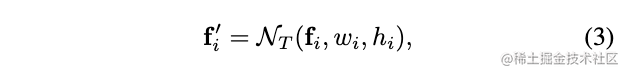$f_i$$i$位置的特征，$(w_i, h_i)$为对应的anchor形状，$\mathcal{N}_T$$3\times 3$可变形卷积，变形卷积的偏移值由$1\times 1$卷积将位置预测分支输出转换获得，$f^{'}_i$为自适应特征，用于后续的anchor调整以及分类预测，具体见图1。

# Training

### Joint objective

网络的整体损失函数由4部分构成，分别是分类损失、回归损失、anchor位置损失以及anchor形状损失：### Anchor location targets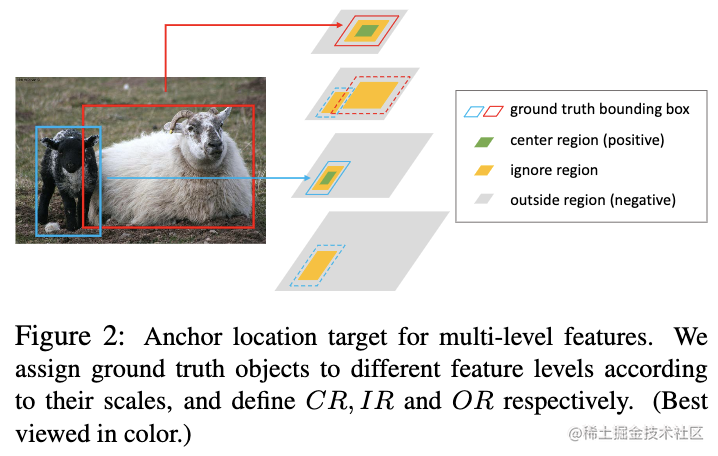假设目标$(x_g, y_g, w_g, h_g)$在特征图上的映射为$(x^{'}_g, y^{'}_g, w^{'}_g, h^{'}_g)$，定义以下三种区域：

• 中心区域$CR=\mathcal{R}((x^{'}_g, y^{'}_g, \sigma w^{'}_g, \sigma h^{'}_g))$，区域内均为正样本点
• 忽略区域$IR=\mathcal{R}(x^{'}_g, y^{'}_g, \sigma_2 w^{'}_g, \sigma_2 h^{'}_g)$\ $CR$$\sigma_2 > \sigma$，区域内的均为忽略点，不参与训练
• 外部区域OR为非IR和CR的区域，区域内均为负样本点

主干网络使用了FPN，FPN的每层应该只负责特定大小范围内的目标的训练。由于相邻层的特征相似，所以将IR区域映射到相邻层，这时的IR区域不考虑CR区域，区域内同样不参与训练，如图2所示。当多个目标重叠时，CR区域优先于IR区域，IR区域优先于OR区域，训练采用Focal loss。

### Anchor shape targets

首先，定义动态anchor $a_{wh}=\{(x_0, y_0, w, h)| w> 0, h > 0\}$与GT间的最优问题为：如果对每个位置都进行公式5的求解，这计算量会相当大，为此，论文用采样的方法来逼近公式5，采样的范围为常见的anchor样例，比如RetinaNet的9种anchor。对于每个位置，选择IoU最大的anchor作为公式5的结果。采样的范围越大，anchor的生成结果更准，但会带来更多的额外计算量，anchor的采用smooth-L1训练：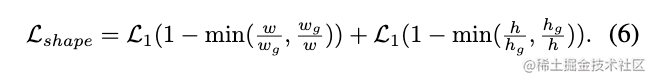# The Use of High-quality Proposals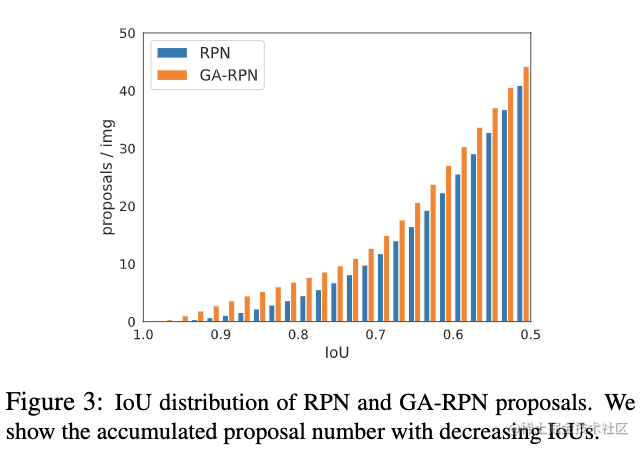将Guided Anchoring嵌入RPN得到增强型GA-RPN，与原版进行对比，从图3可以看到：

• GA-RPN的正样本数量更多
• GA-RPN的高IoU候选框数量更多

从结果来看，GA-RPN的效果比RPN好很多，论文直接将RPN替换GA-RPN仅带来小于1的AP提升。根据观察发现，使用高质量候选框的前提是，需要根据候选框的分布来调整训练数据的分布。所以，在使用GA-RPN时，需要设置更高的正负样本阈值，让网络更加关注高质量的候选框。另外，论文发现GA-RPN也可以fine-tune的形式boost两阶段检测器的性能，给定训练好的检测模型，将RPN替换为GA-RPN进行几轮迭代，能带来不错的性能提升。

# Experiments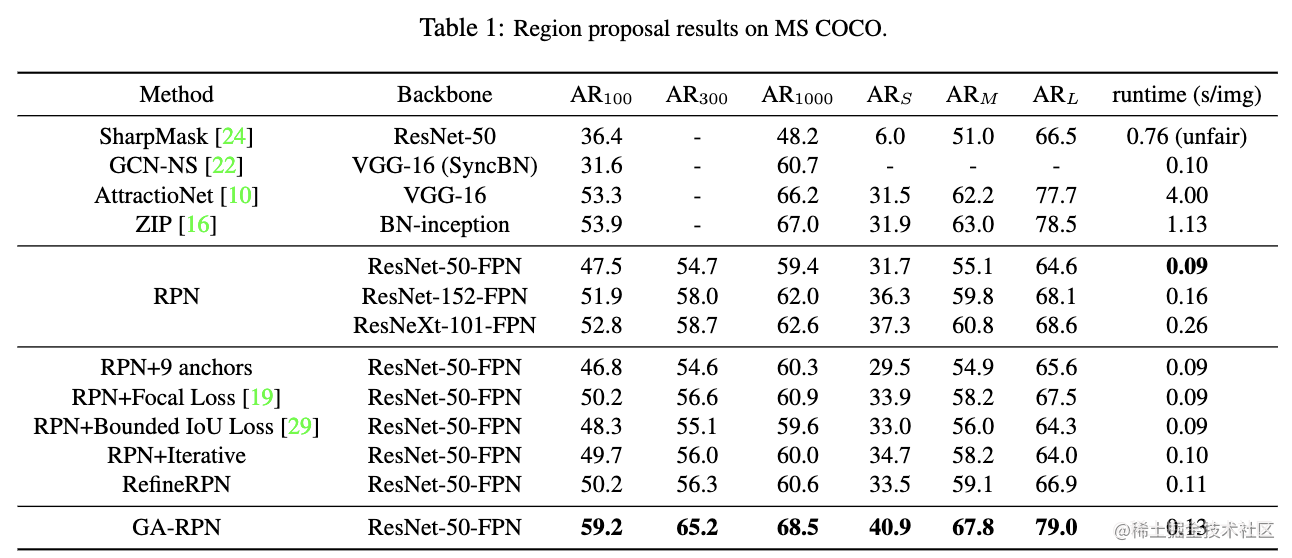与各种候选框方法进行对比。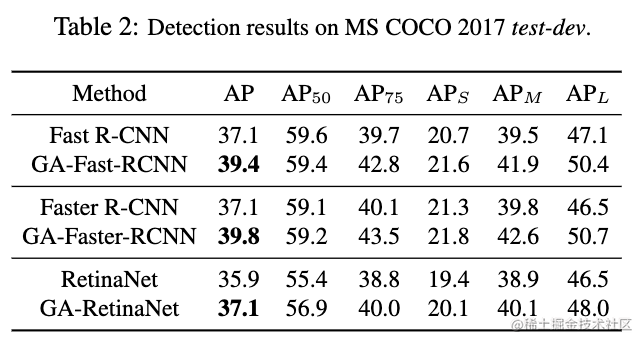嵌入效果对比。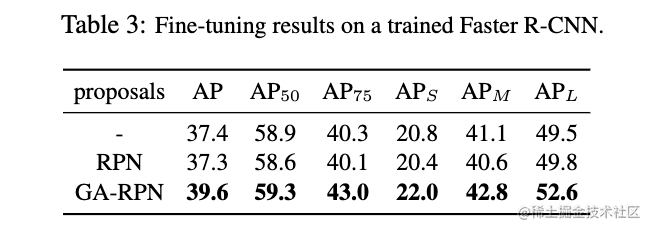fine-tune对比。

# Conclusion

Guided Anchoring通过在线生成anchor的方式解决常规手工预设anchor存在的问题，以及能够根据生成的anchor自适应特征，在嵌入方面提供了两种实施方法，是一个很完整的解决方案。但有个不好的点在于anchor target的生成，为了保证性能弄得不够完美，希望有人能提出更准确且高效的公式5解决方案。